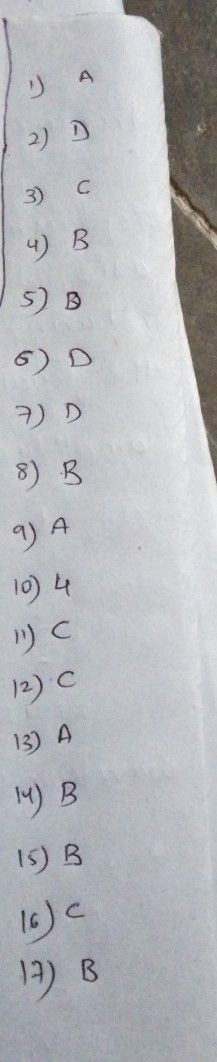Symbol
Problem1. Which A.2x + o5 f = th1e 1 $\left(olloming$ is a quadratic equation? C. x2 1 B.+ 6 = 3 D. x-x = 4 2. In the equation ax2 + bx + c= 0, which of the following cannot be equal to zero for the equationto be quadratic? A a B. b C. c D. a, b, and c 3. Which of the following equations is written in standard form? A.x - 5 =x C. 3x2 + 4x - 5 = 0 B.x2 = 9 D.x2 - x = 6 4. In the equation 8x + 3x² = -5, what is the real number c ? A. -5 B. 5 C. 3 D.8 5. In the equation x2 = 4, what is the real number b ? A. O B. 4 C. 1 D.undefined 6. When the value of b2 - 4ac is greater than zero and perfect square, the roots are A. Real numbers and equal C. rational numbers and not equal B. Irrational and not equal D. no real roots 7. When the value of b2 - 4ac is less than zero, the roots are A. Real numbers and equal C. rational numbers and not equal B. Irrational and not equal D. no real roots 8. How many real roots does the quadratic equation x2 = 25 has? A. One B. two C. three D. no real roots 9. What is the nature of the roots of a quadratic equation if the value of its discriminant is zero? A. The roots are not real. C. The roots are rational and not equal B. The roots are irrational and not equal.D. The roots are rational and equal. 10. What is the sum of the roots of the roots of the quadratic equation x2 + 6x $-14=0$ A. -7 B. -6 C.-3 4. 14 11. If x1 and x2 are the roots of the quadratic equation $ax^{2+bx+c}=0,then$ x1. X2 is A.b/c B. cla C. -b/a D. -c/a 12. If the quadratic equation is $x^{2}-3x=10$ what is the sum of its roots? A. -3 B. -3 C.-10 D. -10 13. In question no. 12, what is the product of the roots? A. - 3 B. -3 $C.-10$ $D$ $x^{2}+x-56=02$ -10 14. What are the roots of the equation $A.$ $2and-1$ $C.-8and7$ $D.3and-2$ B. $8and-7$ 15. Find the $quadratic$ equation whose roots are -5 and 3? A. $x^{2+2x-15}=0$ $C.x^{2-2x-15}=0$ $D.x^{2-2x-15}=0$ $B.$ $x^{2-2x+15}=0$ $-3x+9=02$ 16. $Nhatisthc$ sum of the roots of $thequadrat10$ -3/2 equation 2x A. 2/3 B. 9/2 17. If the $quadxati9$ equation is $C3/2$ $D$ $36x^{2+36x}=-52$ $c$ $k$ $D$ A. -3/7 $B.5/36$ 7/9
7th-9th grade
Calculus
Search count: 113
SolutionQanda teacher - sathish